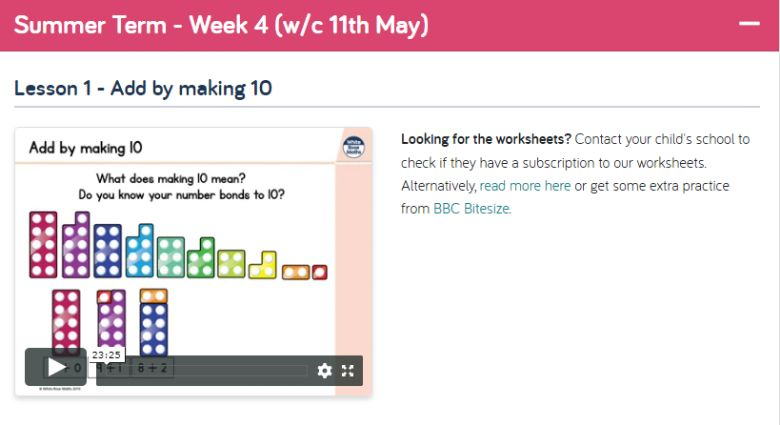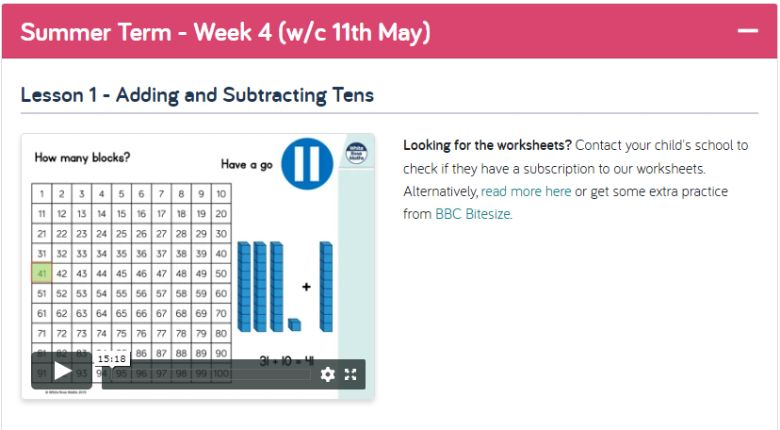Maths

Warm up Activities:-

Numbots

https://play.numbots.com/#/account/search-school

Times Table Rock Stars

https://ttrockstars.com/

Maths Attack

Number of the Week  Represent the number of the week in different ways and see how many calculation you can write that give the answer of that number. See if you can do this systematically.

This week’s number is 38

Main Activity:

White Rose Lessons 1 to 4, watch the video clips (on the link below) and then answer the questions on the sheets below.

https://whiterosemaths.com/homelearning/year-1/L1 - Add by Making 10

L2- Subtract within 10

L3- Add and Subtract Worded Problems

L4 - Compare Number Sentences

L5 - Number of the Week - 38

https://whiterosemaths.com/homelearning/year-2/L1 - Add and Subtract Tens